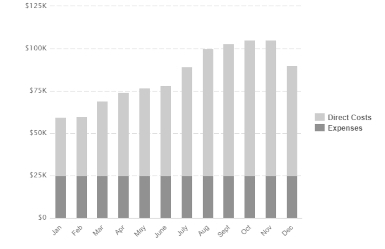# Financial Planning for Construction Projects: Advanced Calculations

Construction projects are complex undertakings that require meticulous financial planning to ensure successful completion within budget and timelines. Advanced calculations play a crucial role in estimating costs, allocating resources, and managing financial risks. In this article, we delve into some key calculations that underpin effective financial planning for construction projects.## 1. Cost Estimation and Budget Allocation

Accurate cost estimation forms the foundation of any construction project's financial plan. Project managers use various methods, including historical data analysis and parametric estimation, to determine the project's overall cost. One essential formula used in cost estimation is the Cost Estimation = (Area × Unit Cost) + Other Costs.

The Unit Cost here refers to the cost per unit area of construction. This can vary based on factors such as location, materials used, and project complexity. Other costs encompass expenses like permits, legal fees, and contingency funds.

## 2. Cash Flow Projection

Cash flow projection is a critical aspect of financial planning, as it ensures that sufficient funds are available throughout the project's duration. The formula for calculating the Net Cash Flow in a given period is:

Net Cash Flow = Cash Inflows - Cash Outflows

Cash inflows include sources like client payments and external funding, while cash outflows encompass labor costs, material procurement expenses, and administrative overheads. By maintaining a positive net cash flow, the project avoids liquidity issues that could lead to delays or interruptions.

## 3. Return on Investment (ROI)

Assessing the project's profitability is essential, and Return on Investment (ROI) is a widely used metric. The ROI formula is:

ROI = (Net Profit / Total Investment) × 100

Here, the Net Profit is the income generated from the project minus all expenses, while the Total Investment includes both initial capital and ongoing costs. A higher ROI indicates a more profitable project, helping stakeholders make informed decisions about resource allocation.

## 4. Depreciation and Amortization

Depreciation and amortization are crucial concepts for accounting and taxation purposes. The formulas for calculating depreciation and amortization are:

Depreciation = (Initial Cost - Salvage Value) / Useful Life

Amortization = Cost / Number of Amortization Periods

Depreciation applies to tangible assets like machinery, where the value decreases over time, while amortization is relevant for intangible assets like patents. Proper calculation of these values helps in accurate financial reporting and tax planning.

## 5. Risk Assessment and Contingency Planning

Risk assessment is integral to financial planning, as construction projects are susceptible to unexpected events. Calculating contingencies involves estimating potential costs and allocating a portion of the budget to address unforeseen issues. The Contingency Reserve can be determined using:

Contingency Reserve = Estimated Project Cost × Contingency Percentage

The Contingency Percentage varies depending on the project's complexity and the level of uncertainty. A higher-risk project will have a higher contingency percentage to mitigate potential financial setbacks.

## 6. Earned Value Analysis

Earned Value Analysis (EVA) is a powerful tool for tracking a project's performance against its planned budget and schedule. The formulas used in EVA include:

Earned Value (EV) = % Complete * Budget at Completion (BAC)

Cost Variance (CV) = EV - Actual Cost (AC)

Schedule Variance (SV) = EV - Planned Value (PV)

These formulas help assess if the project is ahead of or behind schedule and whether it is under or over budget. They enable proactive management to address any deviations from the initial plan.

## 7. Discounted Cash Flow Analysis

For long-term construction projects, Discounted Cash Flow (DCF) analysis is crucial for evaluating the project's financial viability. The Net Present Value (NPV) is calculated using:

NPV = Σ (Cash Flow / (1 + Discount Rate)^n)

Where n is the time period and the Discount Rate represents the cost of capital. A positive NPV indicates the project's potential to generate returns higher than the required rate of return.

## 8. Breakeven Analysis

Understanding the point at which a construction project starts generating profits is essential. Breakeven Analysis helps identify this point and is calculated using the formula:

Breakeven Point = Fixed Costs / (Unit Price - Variable Cost)

Here, Fixed Costs represent the non-variable expenses, Unit Price is the price at which the project is sold, and Variable Cost is the cost incurred per unit of production. The result provides insights into how many units need to be sold to cover all costs.

## 9. Sensitivity Analysis

Construction projects are subject to various uncertainties. Sensitivity Analysis assesses the project's sensitivity to changes in variables like costs, sales prices, and interest rates. By altering these variables and observing their impact on financial metrics, stakeholders can make informed decisions to mitigate risks.

## 10. Monte Carlo Simulation

Monte Carlo Simulation is a powerful technique for risk assessment in construction projects. By simulating a large number of scenarios with varying inputs, it provides a distribution of potential outcomes. This helps in quantifying risks, estimating probabilities of success, and optimizing resource allocation.

In conclusion, financial planning for construction projects involves a range of advanced calculations that go beyond simple cost estimation. These calculations, from cost estimation and cash flow projection to ROI analysis and risk assessment, enable project managers and stakeholders to make informed decisions, allocate resources effectively, and ensure the successful completion of complex construction projects.

Remember, every construction project is unique, and while these calculations provide a strong foundation, it's crucial to adapt and tailor them to the specific circumstances of each project to achieve optimal results.